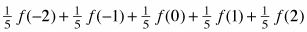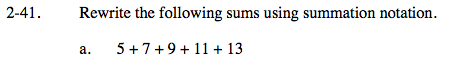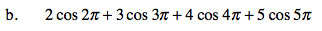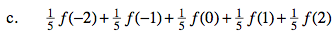### Home > CALC > Chapter Ch2 > Lesson 2.1.3 > Problem2-41

2-41.
1. Rewrite the following sums using summation notation. Homework Help ✎

1. 5 + 7 + 9 + 11 + 13

2. 2 cos 2π + 3 cos 3π + 4 cos 4π + 5 cos 5π

3.$\sum_{n=2}^{5}$